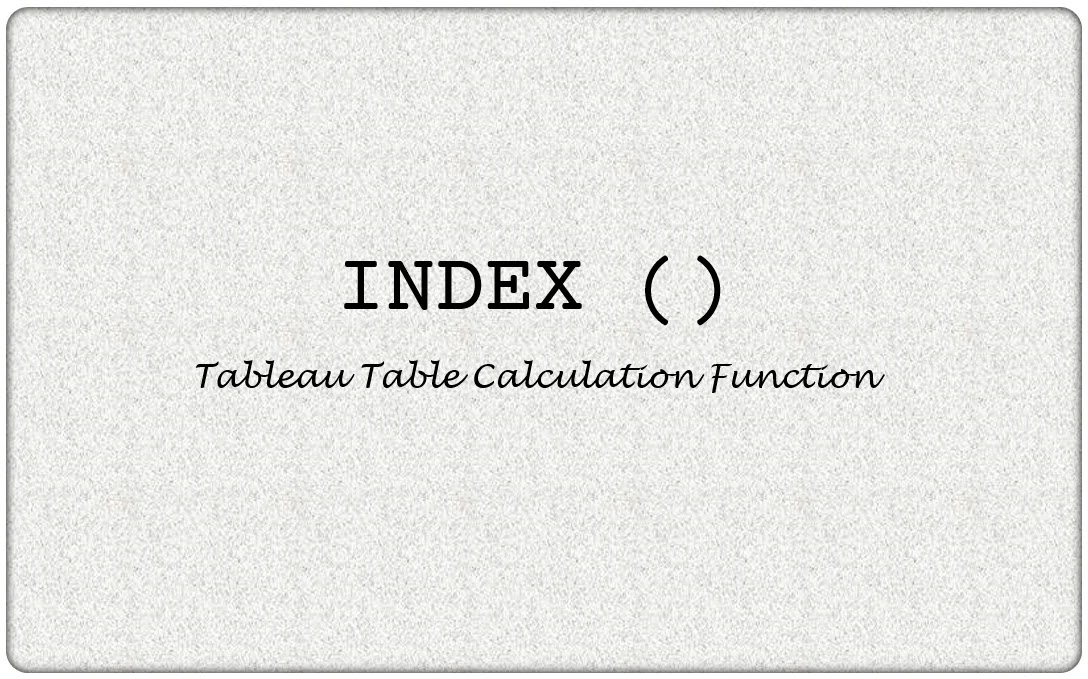# Tableau INDEX Function

×

## Definition

INDEX is a Tableau Table Calculation function. It returns the index of the current row in the partition, without any sorting with regard to value.×

## How to use INDEX function

The INDEX function returns sequentially incrementing values for each row in the partition. The output of the below example is 1 for the first row, 5 for the fifth row and so on. INDEX ()
×

## Use INDEX function in a formula

We can see an use case of INDEX function here in a Radial Bar Chart. It helps create the X axis. The detailed steps can be seen in the Unique Charts section at Learn Tableau. COS ( [ INDEX() - 1 ] * [ PI ] / 180 * [Step Size] ) * [ Rank ]
×

## Example of INDEX in Tableau

INDEX function returns the value of the row with sequentially incrementing values as seen below. Sorting the values by other available measures in the view do not affect the INDEX order.

×